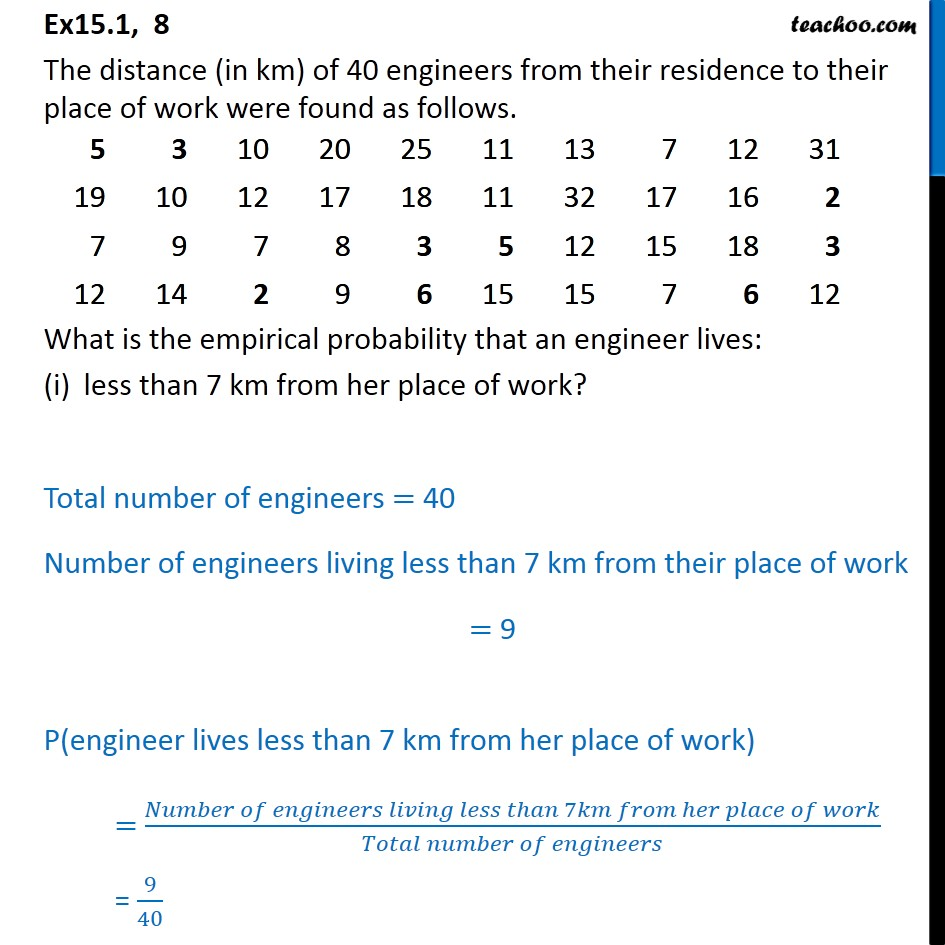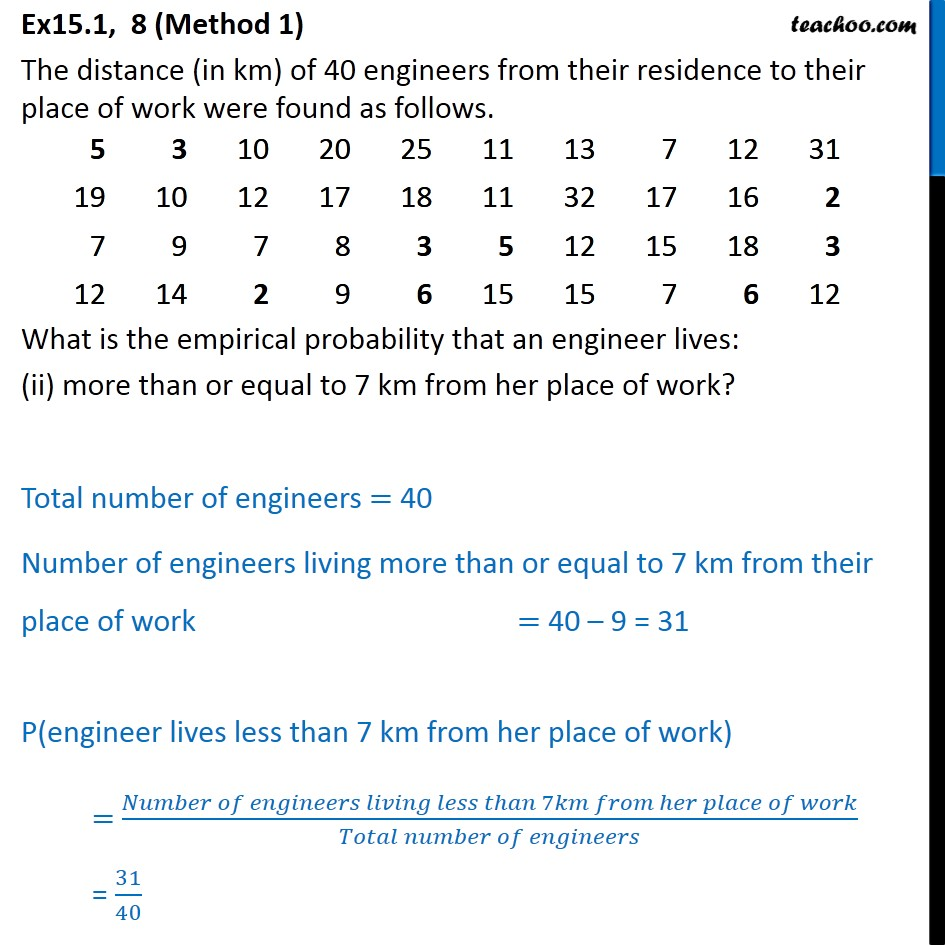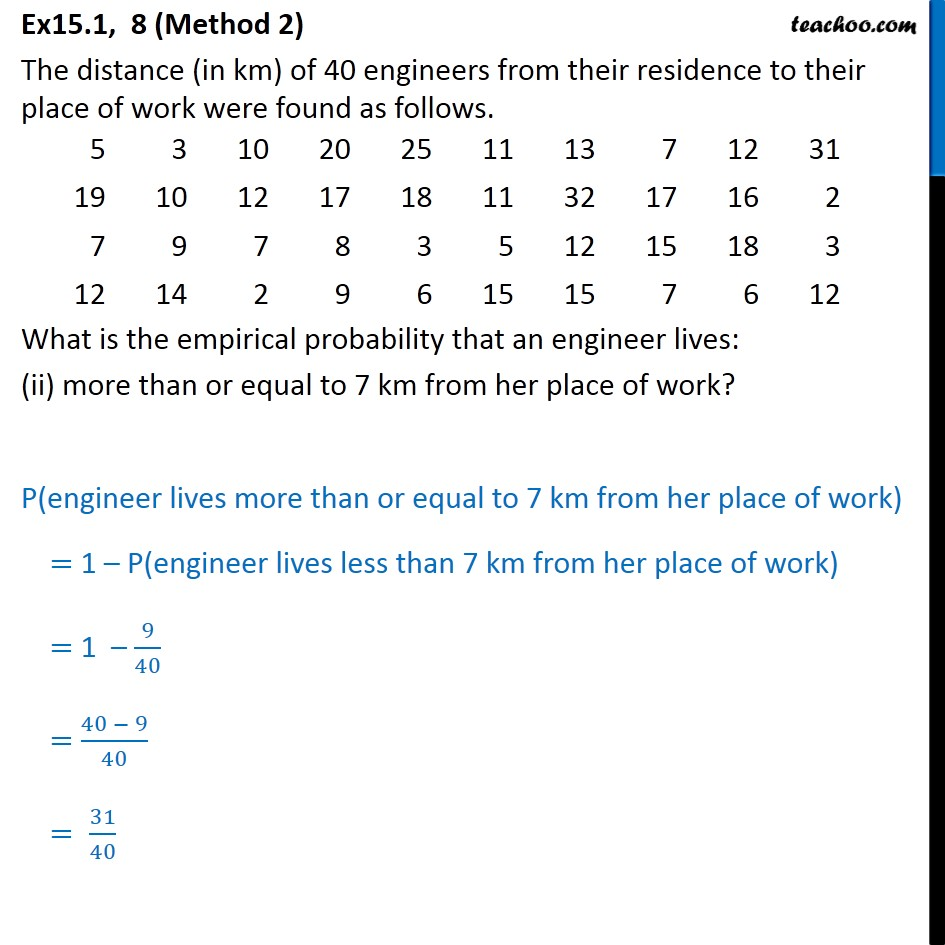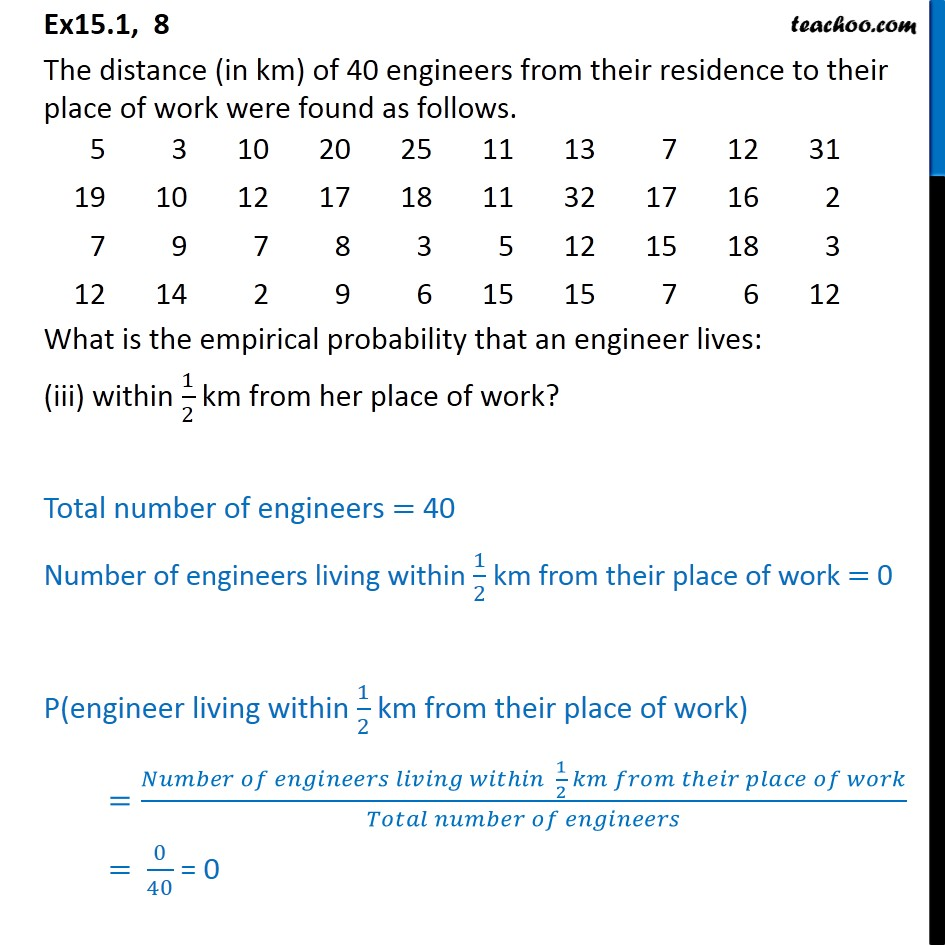Questions - Basic Probability

Probability
Serial order wise
• Questions - Basic Probability

• ExamplesLearn in your speed, with individual attention - Teachoo Maths 1-on-1 Class

### Transcript

Ex15.1, 8 The distance (in km) of 40 engineers from their residence to their place of work were found as follows. What is the empirical probability that an engineer lives: less than 7 km from her place of work? Total number of engineers = 40 Number of engineers living less than 7 km from their place of work = 9 P(engineer lives less than 7 km from her place of work) = (𝑁𝑢𝑚𝑏𝑒𝑟 𝑜𝑓 𝑒𝑛𝑔𝑖𝑛𝑒𝑒𝑟𝑠 𝑙𝑖𝑣𝑖𝑛𝑔 𝑙𝑒𝑠𝑠 𝑡ℎ𝑎𝑛 7𝑘𝑚 𝑓𝑟𝑜𝑚 ℎ𝑒𝑟 𝑝𝑙𝑎𝑐𝑒 𝑜𝑓 𝑤𝑜𝑟𝑘)/(𝑇𝑜𝑡𝑎𝑙 𝑛𝑢𝑚𝑏𝑒𝑟 𝑜𝑓 𝑒𝑛𝑔𝑖𝑛𝑒𝑒𝑟𝑠) = 9/40 Ex15.1, 8 (Method 1) The distance (in km) of 40 engineers from their residence to their place of work were found as follows. What is the empirical probability that an engineer lives: (ii) more than or equal to 7 km from her place of work? Total number of engineers = 40 Number of engineers living more than or equal to 7 km from their place of work = 40 – 9 = 31 P(engineer lives less than 7 km from her place of work) = (𝑁𝑢𝑚𝑏𝑒𝑟 𝑜𝑓 𝑒𝑛𝑔𝑖𝑛𝑒𝑒𝑟𝑠 𝑙𝑖𝑣𝑖𝑛𝑔 𝑙𝑒𝑠𝑠 𝑡ℎ𝑎𝑛 7𝑘𝑚 𝑓𝑟𝑜𝑚 ℎ𝑒𝑟 𝑝𝑙𝑎𝑐𝑒 𝑜𝑓 𝑤𝑜𝑟𝑘)/(𝑇𝑜𝑡𝑎𝑙 𝑛𝑢𝑚𝑏𝑒𝑟 𝑜𝑓 𝑒𝑛𝑔𝑖𝑛𝑒𝑒𝑟𝑠) = 31/40 Ex15.1, 8 (Method 2) The distance (in km) of 40 engineers from their residence to their place of work were found as follows. What is the empirical probability that an engineer lives: (ii) more than or equal to 7 km from her place of work? P(engineer lives more than or equal to 7 km from her place of work) = 1 – P(engineer lives less than 7 km from her place of work) = 1 – 9/40 = (40 − 9)/40 = 31/40 Ex15.1, 8 The distance (in km) of 40 engineers from their residence to their place of work were found as follows. What is the empirical probability that an engineer lives: (iii) within 1/2 km from her place of work? Total number of engineers = 40 Number of engineers living within 1/2 km from their place of work = 0 P(engineer living within 1/2 km from their place of work) =(𝑁𝑢𝑚𝑏𝑒𝑟 𝑜𝑓 𝑒𝑛𝑔𝑖𝑛𝑒𝑒𝑟𝑠 𝑙𝑖𝑣𝑖𝑛𝑔 𝑤𝑖𝑡ℎ𝑖𝑛 1/2 𝑘𝑚 𝑓𝑟𝑜𝑚 𝑡ℎ𝑒𝑖𝑟 𝑝𝑙𝑎𝑐𝑒 𝑜𝑓 𝑤𝑜𝑟𝑘)/(𝑇𝑜𝑡𝑎𝑙 𝑛𝑢𝑚𝑏𝑒𝑟 𝑜𝑓 𝑒𝑛𝑔𝑖𝑛𝑒𝑒𝑟𝑠) = 0/40 = 0